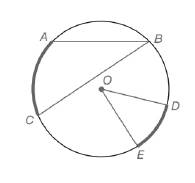Chapter 6.1, Problem 6EElementary Geometry For College St...

7th Edition
Alexander + 2 others
ISBN: 9781337614085

Solutions

Chapter
SectionElementary Geometry For College St...

7th Edition
Alexander + 2 others
ISBN: 9781337614085
Textbook Problem

For Exercises 1 to 8, use the figure provided.Exercises 1-8If m ∠ O = 48.3 ∘ ,  find m D E ⌢ .

To determine

To calculate:

The mDE, if mO=48.3 by using the provided figure.

Explanation

Given:

The provided figure is

Postulate used:

Central angle postulate:

In a circle, the degree measure of a central angle is equal to the degree measure of its intercepted arc.

Calculation:

To find: mDE.

Given that mO=48

Still sussing out bartleby?

Check out a sample textbook solution.

See a sample solution

The Solution to Your Study Problems

Bartleby provides explanations to thousands of textbook problems written by our experts, many with advanced degrees!

Get Started

In Exercises 49-62, find the indicated limit, if it exists. 61. limx1x1x3+x22x

Applied Calculus for the Managerial, Life, and Social Sciences: A Brief Approach

True or False: If f′(x) = g′(x), then f(x) = g(x).

Study Guide for Stewart's Single Variable Calculus: Early Transcendentals, 8th

True or False: The function f is continuous at (0, 0), where

Study Guide for Stewart's Multivariable Calculus, 8th